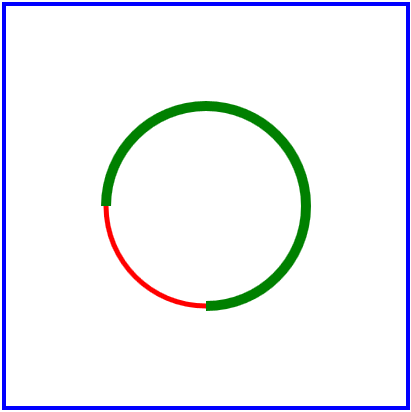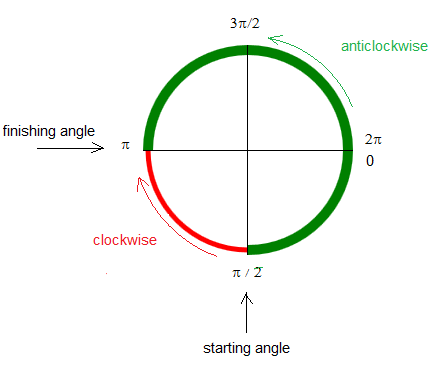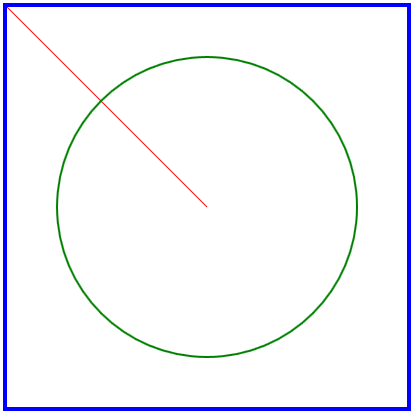# Draw Arcs in HTML5 Canvas

JavaScript and HTML 5 are used to draw arcs and the starting and finishing angles are explained.

## Draw an Arc

Copy and paste the code below in Notepad++ (or any other HTML5 editor) and run it.

<!DOCTYPE html>
<html>
<body>
<canvas id="canvasShapes" width="400" height="400" style="border:4px solid #0000ff;">
This browser does not support the HTML5 canvas tag. </canvas>
<script>

var canvas = document.getElementById("canvasShapes");
var ctx = canvas.getContext("2d");

// draw an arc
ctx.beginPath();
ctx.strokeStyle = "red";
ctx.lineWidth = 5;
ctx.arc(200,200,100,Math.PI/2,Math.PI);
ctx.stroke();

// draw an arc
ctx.beginPath();
ctx.strokeStyle = "green";
ctx.lineWidth = 10;
ctx.arc(200,200,100,Math.PI/2,Math.PI,true);
ctx.stroke();

</script>
</body>
</html>

Explanations

The two statements ctx.arc(200,200,100,Math.PI/2,Math.PI); and ctx.arc(200,200,100,Math.PI/2,Math.PI,true); , included in the program above, are used to draw two arcs whose starting and finishing angles are the same but whose directions are different.

The method ctx.arc(xCenter,yCenter,R,startingAngle,finishingAngle); is used to draw an arc of a circle with radius R and a center at the point (xCenter,yCenter). The starting and ending angle of the arc define the size of the central angle of the arc. The direction of rotation from the starting angle to the finishing is by default a clockwise direction. If true is added as a parameter as in the statement ctx.arc(200,200,100,Math.PI/2,Math.PI,true);, the direction of rotation is counterclockwise as shown in figures 1 and 2 below..
Figure 1.
Figure 2

## Draw a Circle

Copy and paste the code below in Notepad++ (or any other HTML5 editor) and run it.

<!DOCTYPE html>
<html>
<body>
<canvas id="canvasShapes" width="400" height="400" style="border:4px solid #0000ff;">
This browser does not support the HTML5 canvas tag. </canvas>

<script>

// Coordinate system in canvas
var canvas = document.getElementById("canvasShapes");
var ctx = canvas.getContext("2d");

// draw a line
ctx.beginPath(); ctx.strokeStyle = "red";
ctx.lineWidth = 1;
ctx.moveTo(200,200);
ctx.lineTo(0,0); // top left corner
ctx.stroke();

// draw a circle
ctx.beginPath();
ctx.strokeStyle = "green";
ctx.lineWidth = 2;
ctx.arc(200,200,150,0,2*Math.PI);

ctx.stroke();

</script>
</body>
</html>.

Explanations

The same method arc() used to draw arcs is used to draw circles with the starting angle equal to 0 and a finishing angle equal to 2π as in the statement ctx.arc(200,200,150,0,2*Math.PI); included in the program above.